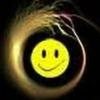BrainDen.com - Brain Teasers
• 0# Playing with infinity: pairing integers with the real numbers

## Question

Cantor tells us that infinities come in "sizes" or cardinalities.

Some infinities are "larger" than others.

The smallest infinite set comprises the counting numbers, 1 2 3 4 5 ...

and any other set that can be put in a 1-1 correspondence with them.

Such infinities are called "countable."

The rational numbers are also countable, but the real numbers are not.

Occasionally someone will attempt to pair the counting number with the reals.

One such scheme is shown below, and the argument goes as follows:

The left column is the endless list of integers in numerical order

The right column contains a decimal fraction formed by reversing the digits and placing a decimal point in front.

Since the left hand list proceeds without limit it eventually contains every possible sequence of digits.

Then the right hand list also catches every sequence of digits and thus represents the real numbers less than unity.

Numbers greater than unity can be constructed by sets with a shift in the position of the decimal point.

The union of two or more sets of equal cardinality has the same cardinality as its component sets.

This correspondence is sufficient to prove the two sets have the same cardinality.

The argument is obviously flawed, and the question is to expose the flaw.

Here are the lists:

Integers Decimal fractions

1 .1

2 .2
3 .3
4 .4
. .
. .
. .
10 .01
11 .11
12 .21
13 .31
. .
. .
. .
100 .001
101 .101
102 .201
103 .301
. .
. .
. .
1234 .4321
. .
. .
. .

## Recommended Posts

• 0

The left hand list only contains the set of terminating decimals, for example it does not contain sqrt(2)/2

##### Share on other sites
• 0

i tend to agree with James. however let's try this construction.

first, I'll use binary, so only 1 and 0.

then I'll construct a transcendental number.

let 0->01 and 1 -> 0

0

01

010

01001

01001010

repeat indefinitely, use this number as our 1.

1 -> 0.01001010..

we know the rational numbers are countably infinite, and doubling the rational numbers is not enough to change countability.

so, i propose we rotate between the list of rational numbers, and xor the list rational numbers with this transcendental number.

prove this doesn't go through every positive number.

Edited by phil1882

##### Share on other sites
• 0

The left hand list only contains the set of terminating decimals, for example it does not contain sqrt(2)/2

Right.

Column 2 is only a subset of the rationals.

##### Share on other sites
• 0

does no one want to take on my challenge ???!!! :-)

##### Share on other sites
• 0

i tend to agree with James. however let's try this construction.

first, I'll use binary, so only 1 and 0.

then I'll construct a transcendental number.

let 0->01 and 1 -> 0

0

01

010

01001

01001010

repeat indefinitely, use this number as our 1.

1 -> 0.01001010..

we know the rational numbers are countably infinite, and doubling the rational numbers is not enough to change countability.

so, i propose we rotate between the list of rational numbers, and xor the list rational numbers with this transcendental number.

prove this doesn't go through every positive number.

Which rationals XOR with 0.01001010... to give e/3 and pi/4?

##### Share on other sites
• 0

i might not be able to find the exact coordinate since 0.01001010 is transcendental, and I'm not quite sure how to map one transcendental number to another, but i can give better and better coordinates depending on your approximation of e/3 and pi/4.

what i can guarantee is that there are numbers that are as random as e/3 and pi/4.

## Join the conversation

You can post now and register later. If you have an account, sign in now to post with your account.×   Pasted as rich text.   Paste as plain text instead

Only 75 emoji are allowed.

×   Your previous content has been restored.   Clear editor

×   You cannot paste images directly. Upload or insert images from URL.

×